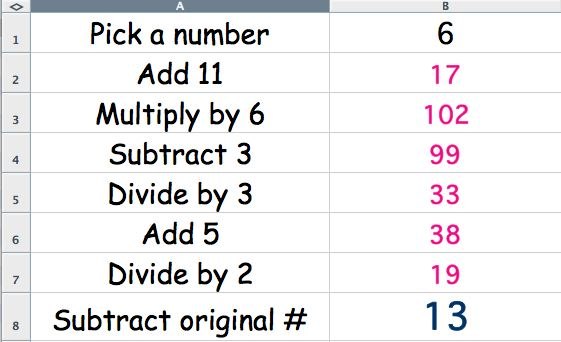The Famous Jinx Puzzle Student's page 2 Revised 6.7.10

The Jinx Calculator
To help you find out if this puzzle will work for all numbers, we will use a special spreadsheet “calculator." Here's what your Jinx Calculator will look when you open it. Notice that 6 was the chosen number and entered in cell B1.The spreadsheet then quickly did all the calculations and the answer was once again 13 - the unlucky number. Can you find a number that will make the puzzle not end in 13 (and not jinx you?) Use the Excel version of the Jinx Calculator to help you.

(Hint: Try different kinds of numbers not just whole numbers.  Like these: 2.5, -56, 3000, 999999999, .000005. Fractions can also be tried. For example if you try 1/2, you enter =1/2 into the cell.

Share a few of your interesting examples of numbers that you used here.

Go to the results page and describe the results.

Questions: